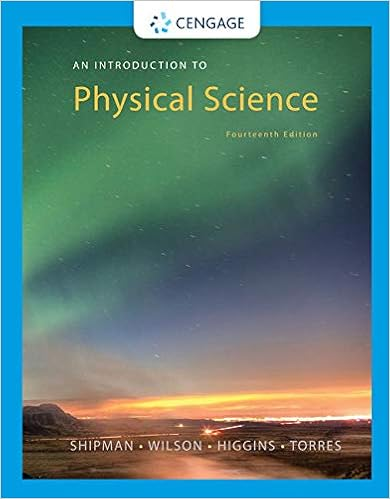# Acceleration of the expansion and dark energy

• Notes
• 18

This preview shows page 9 - 11 out of 18 pages.

##### We have textbook solutions for you!
The document you are viewing contains questions related to this textbook.The document you are viewing contains questions related to this textbook.
Chapter 18 / Exercise 11
An Introduction to Physical Science
Shipman/WilsonExpert Verified
Acceleration of the Expansion and Dark Energy Although we do not understand why the universe is expanding, we do know from General Relativity that gravity should counter that expansion. This is because mass bends space-time, while space-time forms the lattice through which masses move. Hence, mass and space-time are tied to each other. For this reason, it is natural to expect that the expansion should have decelerated as the universe aged. It is actually possible to determine directly whether this is the case. When we observe distant galaxies, we are looking back in time, since the light that we detect left that galaxy long before it reached us after traveling across space. If the expansion has been slowing down, then the value of the slope of the velocity vs . distance graph should be higher at very large distances corresponding to times closer to the beginning of the Big Bang. That is, if the rate of expansion were higher in the past, the graph would curve up rather than remain a straight line. In this case, Hubble’s “constant” would not really be constant. Figure 11-7. The curvature of a sphere or circle is only apparent when the radius is not many of orders of magnitude larger than we can see. Note how the section of the circle inside the square becomes straighter as the radius of the circle increases. According to current cosmological theory, Inflation did the same to the universe, making space-time very flat.
##### We have textbook solutions for you!
The document you are viewing contains questions related to this textbook.The document you are viewing contains questions related to this textbook.
Chapter 18 / Exercise 11
An Introduction to Physical Science
Shipman/WilsonExpert Verified
Chapter 11: Big Bang Cosmology 11-10 Actually, when we observe extremely distant galaxies it is no longer convenient — or even correct — to use velocity on the Hubble Law graph (Fig. 10-2). Rather, astronomers usually plot on the horizontal axis the redshift z , which is defined in equation (10-3). One plus the redshift (1+ z ) (see eq. 10-2) corresponds to how many times the universe has expanded since the light we now observe left the galaxy that emitted it. For example, for a galaxy at a redshift z =3 (so 1+ z = 4), the universe has expanded by 4 times during the time it took for the light to travel from the galaxy to us. On the vertical axis astronomers often plot the brightness of the object, which is the quantity that they measure when they determine the distance through use of equation (6-2). Figure 11-8 shows the graph of the brightness of a number of a certain type of exploding star called a Type Ia supernova vs. redshift z . Although the data points are somewhat scattered in the crucial upper right-hand corner, the observations agree better with the case in which the expansion of the universe is accelerating . What could cause the expansion of the universe to accelerate? There must be some property of the universe or some force that is stronger than the combined gravity of all the matter in the universe. Recall from Chapter 10 that Einstein included such a property, which he called the Cosmological constant , in General Relativity. This would need to have strange properties to remain constant while the volume of the universe increases. An alternative, nicknamed “ether,” is some hypothetical property of space that would
•••